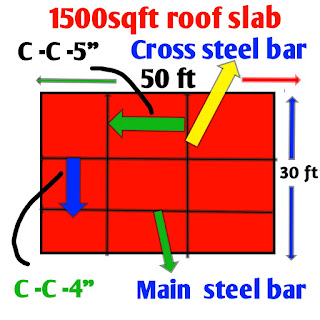# Quantity of cement sand and aggregate for1500 sq ft slab

Quantity of cement sand and aggregate for 1500 square feet slab and construction cost of RCC slab in India and cost per square feet concrete slab in IndiaQuantity of cement sand and aggregate for 1500 sq ft slab Roof slab

quantity of cement sand and aggregate  for 1500 square feet slab ,for the roof cast of 1500 square feet building roof slab, you have require Steel bar ,sand, cement and aggregate ,how we calculate quantity of cement, sand, Steel bar and aggregate required ,you know that for the casting of roof slab we used M20 mix concrete.

## What is M-20 mix grade of Concrete

quantity of cement sand and aggregate for 1500 square feet slab,
In M20 mix concrete M is stand for mix and numerical figure 20 is stand for characteristic of compressive strength 20N/mm2 after mixing and casting of roof slab.

quantity of material in slab formation it calculated by M20 mix in which ratio of sand cement and aggregate is 1: 1.5 :3

●M-mix Ratio :-1 : 1.5 : 3 in which one part is cement 1. 5 part is sand and 3 part is aggregate

## Construction cost of RCC slab in India

For construction cost of RCC slab in India we have to find out quantity of steel, cement bags, fine aggregate and coarse aggregate are required for roof casting of 1500 square feet 5 inch slab

### How to calculate Steel for RCC slab

Steel bar calculation:- In roof slab there is a two types of Steel bar used main bar and cross bar:-

1) Main bar:- it is also called as shortist span bar usually used at bottom of slab having spacing 4 inch between the two bar, main bar used in shorter direction having 10 mm dia of Steel bar.

2) Cross bar:- it is also called as longer span bar or distribution bar mainly used at top of the main bar, cross bar usually dia of 8mm Steel bar used in longer direction.

Given dimensions:-

Thickness of slab=5″

Length of slab =50′

Area of slab =50’×30’=1500 sqft

M -20 -mix =1:1.5:3 (concrete)Quantity of sand cement and aggregate for 1500 sq ft slab Roof slab

●Volume Calculation:

1) wet volume=Area × thicknessof slab

Vw= 1500 sqft× 5′
Vw= 1500sqft×5/12′
Vw =625 cft

If you want to convert it into cubic metre you should divide by 35.3147
1m3 =35.3147 cft

Wet volume=625/35.3147=17.698 m3

2) Dry volume:

we know that wet volume have water and bubbles it can be remove by vibrator or compressor machine so more quantity of material is required hence dry volume is increased by 54 percent of wet volume , so we can multiply 1.54 in wet volume to calculate dry volume

Vd =1.54 ×17.698 m3
Dry volume=27.255 m3

●Weight calculation of Steel:- quantity of steel required in roof slab is about 0.5℅ to 1% of dry volume then average is 0.75% of dry volume

Weight =0.75%of dry volume×density of steel
Density of steel is=7850 kg/m3

W =0.75/100×27.255m3 ×7850kg/m3
Weight=1602.8 kg

So  weight of Steel bar is require for 1500 square feet Roof slab= 1602.8kg
If Market rate of steel bar is 60 rupees per kg then

total cost of Steel bar =1602.8×rs 60
Total cost= Rs 96172

### How much quantity of sand required for  Roof cast  of rcc slab

In M20 ratio of sand cement and aggregate is 1:1.5:3 ,in this ratio 1.5 part is sand
Total proportion =1+1.5+3=5.5

Volume of sand in cft
Volume =1.5 /5.5×27.225×35.3147 cft

Volume of sand =262.21 cft

If market rate of 80 rupees per CFT then total cost of quantity of sand

Total cost =rs80×262.21
=Rs 20976

### How much cement is required for roof cast of 1500 square feet RCC slab

●Cement calculation:- in M20 mix 1 part is cement quantity

Weight of cement=1/5.5×dry volume in m3×density of cement
Density of cement=1450kg/m3

Weight =1/5.5×27.255 m3×1450 kg/m3
=7177.5 Kg
1 bag cement =50kg
No.of bags =7177.5/50

=143.55 Bags cement

If market rate of cement is 350 rupees per bags then total cost

Total cost =143.55 ×rs 350

= Rs 50242

### How much course aggregate is required for 1500 RCC roof slab

●Aggregate Calculation:-

in M20 mix there is 3 part of aggregate quantity

Volume of aggregate=3/5.5×dry volume in CFT
Volume =3/5.5×27.255×35.3147 cft

Volume =524cft

If market rate of aggregate is is 70 rupees per CFT then total cost of aggregate

Total cost =rs 70×524
Rs=rs 36709

### total cost of material required for 1500 square feet RCC slab

Cost of steel=rs 96172
Cost of cement=rs 50242
Cost of sand=rs 20976
Cost of stone=rs 36709
Labour charge and machine cost =rs 75000 (approx)

Total cost=Rs 279099

Hence total cost of roof slab of 1500 square feet building require rs 279099

1602.8 Kg steel, 262.21 Cuft sand, 143.55 bags ( 7177.5 kg) cement and 524 Cuft aggregate is required for roof cast of 1500 square feet rcc slab and total cost of material is about rs 279099.